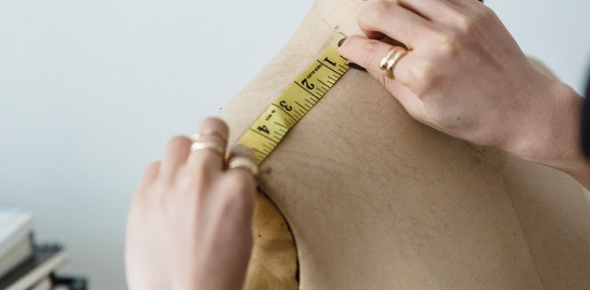# Multiple Choice Questions On Metric Measures For Grade 5

10 Questions | Attempts: 1791
ShareSettingsIf you're looking for multiple-choice questions based on metric measures for grade 5th students, you are at the right place. The quiz below consists of mathematics measurements questions and answers that will help students practice metric system questions. The metric system is a unit of measurement to calculate an object's mass, distance, and volume. Do you know how many centimeters are there in one meter? Give this quiz a try and learn many interesting things. Good luck, buddy!

• 1.
1m=______cm.
• A.

1000

• B.

10

• C.

100

• D.

10000

• 2.
7400cm=_____m
• A.

740

• B.

74

• C.

7400

• D.

740000

• 3.
6m50cm=_____cm.
• A.

6050

• B.

650

• C.

6500

• D.

60500

• 4.
40mm=______cm.
• A.

4

• B.

500

• C.

5000

• D.

50000

• 5.
26m=______cm.
• A.

206

• B.

260

• C.

2600

• D.

26000

• 6.
1mile=______Kilometre
• A.

1.5

• B.

1.9

• C.

1.8

• D.

1.6

• 7.
9km=______m
• A.

90

• B.

900

• C.

9000

• D.

90000

• 8.
5000m=______km.
• A.

5

• B.

50

• C.

500

• D.

50000

• 9.
200cm+400cm=______cm.
• A.

500

• B.

700

• C.

800

• D.

600

• 10.
1km=______m.
• A.

10

• B.

1000

• C.

100

• D.

1000

## Related TopicsBack to top
×

Wait!
Here's an interesting quiz for you.